Courses

WBJEE Maths Test - 3

75 Questions MCQ Test WBJEE Sample Papers, Section Wise & Full Mock Tests | WBJEE Maths Test - 3

Description
Attempt WBJEE Maths Test - 3 | 75 questions in 120 minutes | Mock test for JEE preparation | Free important questions MCQ to study WBJEE Sample Papers, Section Wise & Full Mock Tests for JEE Exam | Download free PDF with solutions
QUESTION: 1

Solution:
QUESTION: 2

Solution:
QUESTION: 3

The coefficient of x3 in ((√x5) + (3/√x3))6 is

Solution:
QUESTION: 4
The radical axis of the circles, belongs to the coaxial system of circles whose limiting points are (1,3) and (2,6) is
Solution:
QUESTION: 5

If a < 0 < b then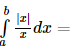Solution:
QUESTION: 6

The centre and radius of the circle with the segment of the line x+y=1 cut of by the coordinate axes as diameter are

Solution:
QUESTION: 7

If (a+ib)(c+id)(e+if)(g+ih) = A+iB, then (a2+b2)(c2+d2)(c2+f2)(g2+h2) =

Solution:
QUESTION: 8
If we express [(cos 2θ - i sin2θ)4(cos4θ + i sin4θ)-5]/[(cos3θ + i sin 3θ)-2(cos3θ - i sin3θ)]-9 in the form of x + iy,we get
Solution:
QUESTION: 9

The area bounded by two curves y2=4ax and x2=4ay is

Solution:
QUESTION: 10

If a, b, c are different and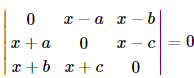then x =

Solution:
QUESTION: 11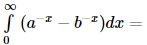Solution:
QUESTION: 12

The solution of the differential equation x{y d2y/dx2 + (dy/dx)2}=y dy/dx is :

Solution:
QUESTION: 13

The orthogonal trajectories of the family y2=4ax+4a2 is the family :

Solution:
QUESTION: 14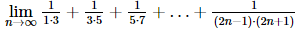Solution:
QUESTION: 15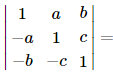Solution:
QUESTION: 16

If siny=x sin (a+y), (dy/dx)=

Solution:
QUESTION: 17

The differential of sin⁻1[(1-x)/(1+x)] w.r.t. √x is equal to

Solution:
QUESTION: 18

The eccentricity of ellipse 4x2 + 9y2 = 36 is

Solution:
QUESTION: 19

The major axis of an ellipse is three times the minor axis, then the eccentricity is

Solution:
QUESTION: 20

The parametric equations of the hyperbola x2/a2 - y2/b2 = 1 are

Solution:
QUESTION: 21

The eccentricity of the conic 9x2 - 16y2 = 144 is

Solution:
QUESTION: 22

Let f(x) = tan-1 {φ(x)}, where φ(x)is monotonically increasing for 0 < x < π/2. Then f(x) is

Solution: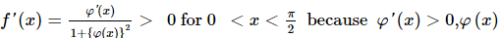being monotonically increasing

QUESTION: 23

tan⁻1(1/4) + tan⁻1(2/9) is equal to

Solution:
QUESTION: 24

If A and B are two matrices such that AB = B and BA = A, then A2 + B2 =

Solution:
QUESTION: 25

A function f(x) is defined as f (x) = [1 − x2] , − 1 ≤ x ≤ 1 , where [x] denotes the greatest integer not exceeding x. The function f(x) is discontinuous at x = 0 because

Solution:
QUESTION: 26

A square tank of capacity 250 cubic m has to be dug out. The cost of land is Rs 50 per sq.m. The cost of digging increases with the depth and for the whole tank is 400 (depth)2 rupees. The dimensions of the tank for the least total cost are

Solution:
QUESTION: 27
If z₁,z₂,z₃ are complex numbers such that |1/z₁+1/z₂+1/z₃|=1, then |z₁+z₂+z₃| is equal to
Solution:
QUESTION: 28

The slope of the normal at the point (at2,2at) of the parabola y2=4ax is

Solution:
QUESTION: 29

The normals to the parabola y2=4ax from the point (5a,2a) are

Solution:
QUESTION: 30
In how many different ways can the letters of the word 'AUCTION' be arranged so that the vowels always come together?
Solution:
QUESTION: 31
In how many ways can the letters of the word 'LEADER' be arranged?
Solution:
QUESTION: 32
Every body in a room shakes hand with every body else. The total number of hand shakes is 66. Then the number of persons in the room is
Solution:
QUESTION: 33
The probability that at least one of the events A and B occurs is 0.6. If A and B occur simultaneously with probability 0.2, then P(A̅) + P(B̅) is
Solution:
QUESTION: 34
A die is thrown 100 times. If getting an even number is considered a success, then the variance of the number of successes is
Solution:
QUESTION: 35
Suppose n≥3 person are sitting in a row. Two of them are selected at random. The probability that they are not together, is
Solution:
QUESTION: 36

If α, β are the roots of the equation x2+ 2x + 4 = 0, then (1/α3) + (1/β3) is equal to

Solution: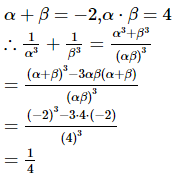QUESTION: 37

In ΔABC , If a2 + b2 + c2 = 8R2 , then the triangle is

Solution:
QUESTION: 38

In ΔABC , if x = tan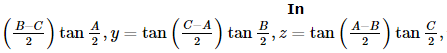then x + y + z in terms of x , y , z only is

Solution:
QUESTION: 39

The equation 3sin2x + 10cosx - 6 = 0 is satisfied, if

Solution:
QUESTION: 40

If f ″ (x)  < 0∀x ∈ (a , b), then f'(x) = 0

Solution:

Suppose, there are two points x1 and x2 in (a,b) such that f'(x1) = f'(x2) = 0. By Rolle's theorem applied to f' on [x1 , x2], there must then be a c ∈ (x1, x2 ) such that f''(c) = 0. This contradicts the given condition f ″ (x)  < 0∀x ∈ (a , b) Hence, our assumption is wrong. Therefore, there can be at most one point in (a,b) at which f'(x) is zero.

QUESTION: 41
If second term of a G.P. is 2 and the sum of its infinite terms is 8, then its first term is
Solution:
QUESTION: 42
The nth term of the series 3, √3, 1,... is 1/243, then n is
Solution:
QUESTION: 43

((1)/(1 x 2)) + ((1/2) x (3)) + ((1/3) x (4)) + ... + ((1)/(n(n + 1))) equals

Solution:
QUESTION: 44
A G.P. consist of an even number of terms. If the sum of all the terms is 5 times the sum of the terms occupying odd places, then the common ratio will be
Solution:
QUESTION: 45

Sin⁻1(3/5) + tan⁻1(1/7) is equal to

Solution:
QUESTION: 46

The set A [x:x ∈ R, x2=16 and 2x=6] equals

Solution:
QUESTION: 47
The equation of a line passing through the intersection of lines 3x-2y-1=0 and x-4y+3=0 and point (π,0) is
Solution:
QUESTION: 48

Points (0,0), (2,-1) and (9,2) are vertices of a triangle, then cosB =

Solution:

Given,

Vertices of a ΔABC are (0,0), (2,-1) and (9,2) are vertices of a triangle, then cosB =

From figure,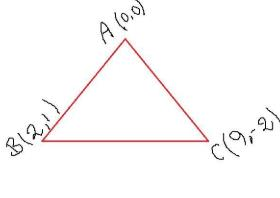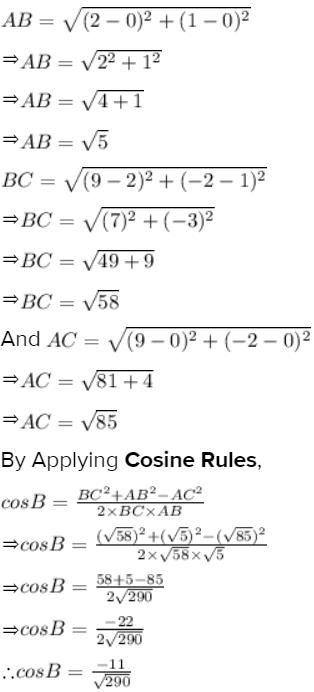QUESTION: 49

The roots of the equation x3 − 2x2 − 4x + 8 = 0 are

Solution: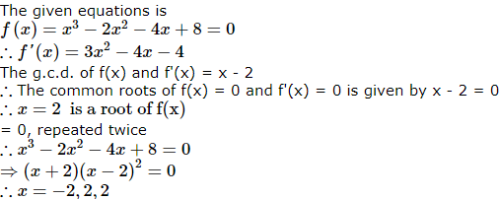QUESTION: 50

If 2cos2x+3sinx-3=0, 0≤x≤180º, then x=

Solution:
QUESTION: 51

From a fixed point A on the circumference of a circle of radius a, the perpendicular AC is drawn to the tangent at B(a variable point). The maximum area of ΔABC is

Solution:
QUESTION: 52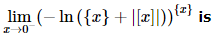(where [.] is the greatest integer function and {.} is the fractional part function)

Solution:
QUESTION: 53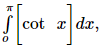where [.] denotes the greatest integer function, is equal to

Solution:
QUESTION: 54

Let R be the real line. Consider the following subsets of the plane RxR.
S={(X,Y):y=x+1 and 0<x<2}, T={(X,Y):x-y is an integer}. Which one of the following is true?

Solution:
QUESTION: 55

If two events A and B are such that P(Ac) = 0.3, P(B) = 0.4 and P A ∩ B c = 0.5, then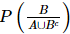is equal to

Solution:
QUESTION: 56

Let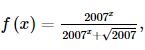then the sum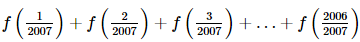is equal to

Solution:
QUESTION: 57

If l is the length of the intercept made by a common tangent to the circle x2 + y2 = 16 and the ellipse x2/25 + y2/4 = 1, on the coordinate axes, then 81l2 + 3 is equal to

Solution:
QUESTION: 58

{x} and [x] represent fractional and integral part of x, then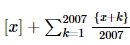is equal to

Solution:
QUESTION: 59

Consider the equation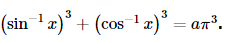The parameter 'a' so that the given equation has a solution which satisfies

Solution:
QUESTION: 60

A bag contains some white and some black balls, all combinations of balls being equally likely. The total number of balls in the bag is 10. If three balls are drawn at random without replacement and all of them are found to be black, the probability that the bag contains 1 white and 9 black balls is

Solution:
QUESTION: 61

A non-zero vector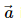is parallel to the line of intersection of the plane determined by the vectors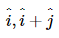and the plane determined by the vectors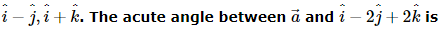Solution:
QUESTION: 62

A line is drawn from A( − 2, 0) to intersect the curve y2 = 4x in P and Q in the first quadrant such that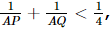then slope of the line is always

Solution:
QUESTION: 63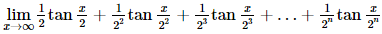is

Solution:
QUESTION: 64

The equation b cos x − cos 2x = 2b − 7 possess a solution if

Solution:
QUESTION: 65

If a = cos 2α + i sin 2α , b = cos 2β + i sin 2β ,
c = cos 2γ + i sin 2γ and d = cos 2δ + i sin 2δ , then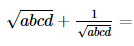Solution:
*Multiple options can be correct
QUESTION: 66

The equation of the tangents drawn from the origin to the circle x2 + y2 − 2rx − 2hy + h2 = 0 , are

Solution:
*Multiple options can be correct
QUESTION: 67

If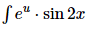dx can be found in terms of known functions of x then u can be

Solution:
*Multiple options can be correct
QUESTION: 68

The number of ways in which we can choose 2 distinct integers from 1 to 100 such that difference between them is at most 10 is

Solution:
*Multiple options can be correct
QUESTION: 69

If the chord y = mx + 1 of the circle x2 + y2 = 1 subtends an angle of measure 45º at the major segment of the circle then the value of m is:

Solution:
*Multiple options can be correct
QUESTION: 70

The set of discontinuities of the function f (x)  = ( 1/2 − cos2x ) contains the set

Solution:
*Multiple options can be correct
QUESTION: 71

In the set R of real numbers the function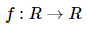is defined such that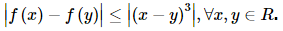is

Solution:
*Multiple options can be correct
QUESTION: 72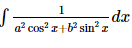is equal to

Solution:
*Multiple options can be correct
QUESTION: 73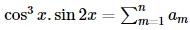sin mx is an identify in x , then

Solution:
*Multiple options can be correct
QUESTION: 74

The interval to which a may belong so that the function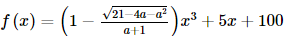increasing ∀× ∈ R

Solution:
*Multiple options can be correct
QUESTION: 75

In a triangle ABC, 3sinA + 4cosB = 6 and 4sinB + 3cosA = 1

Solution:Use Code STAYHOME200 and get INR 200 additional OFF Use Coupon Code

Track your progress, build streaks, highlight & save important lessons and more!

Similar ContentRelated tests